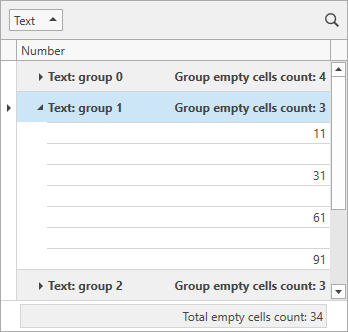# SummaryItemBase.SummaryType Property

Gets or sets the aggregate function type. This is a dependency property.

Namespace: DevExpress.Xpf.Grid

Assembly: DevExpress.Xpf.Grid.v22.2.Core.dll

NuGet Package: DevExpress.Wpf.Grid.Core

## Declaration

``public SummaryItemType SummaryType { get; set; }``

## Property Value

Type Description
SummaryItemType

A SummaryItemType enumeration value that specifies the aggregate function type.

Available values:

Name Description
Sum

The sum of all values in a column.

Min

The minimum value in a column.

Max

The maximum value in a column.

Count

The record count.

Average

The average value of a column.

Custom

Specifies whether calculations should be performed manually using a specially designed event.

None

Disables summary value calculation.

## Remarks

There are five predefined aggregate functions. These are: Sum, Min, Max, Average and Count. To implement your own aggregate function, set the SummaryType property to SummaryItemType.Custom, and handle the GridControl.CustomSummary event.

Average and Sum summaries are only calculated for columns that contain numerical data.

## Example

The following example demonstrates how to use custom summaries to count the total number of empty cells in the specified grid column:``````<dxg:GridControl x:Name="grid"
CustomSummary="OnCustomSummary">
<dxg:GridControl.Columns>
<dxg:GridColumn FieldName="Text" GroupIndex="0" />
<dxg:GridColumn FieldName="Number" />
</dxg:GridControl.Columns>
<dxg:GridControl.View>
<dxg:TableView x:Name="view"
AutoWidth="True"
TotalSummaryPosition="Bottom" />
</dxg:GridControl.View>
<dxg:GridControl.TotalSummary>
<dxg:GridSummaryItem DisplayFormat="Total empty cells count: {0}"
FieldName="Number"
SummaryType="Custom" />
</dxg:GridControl.TotalSummary>
<dxg:GridControl.GroupSummary>
<dxg:GridSummaryItem DisplayFormat="Group empty cells count: {0}"
FieldName="Number"
SummaryType="Custom" />
</dxg:GridControl.GroupSummary>
</dxg:GridControl>
``````
``````void OnCustomSummary(object sender, CustomSummaryEventArgs e) {
if(((GridSummaryItem)e.Item).FieldName != "Number")
return;
if(e.SummaryProcess == CustomSummaryProcess.Start) {
e.TotalValue = 0;
}
if(e.SummaryProcess == CustomSummaryProcess.Calculate) {
if(IsEmptyCell(e.FieldValue))
e.TotalValue = (int)e.TotalValue + 1;
}
}
bool IsEmptyCell(object fieldValue) {
return !((int?)fieldValue).HasValue;
}
``````

The following code snippets (auto-collected from DevExpress Examples) contain references to the SummaryType property.

Note

The algorithm used to collect these code examples remains a work in progress. Accordingly, the links and snippets below may produce inaccurate results. If you encounter an issue with code examples below, please use the feedback form on this page to report the issue.